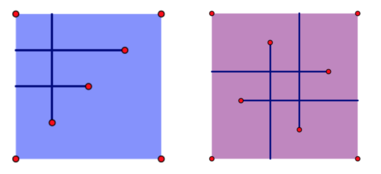o
osc_wws45aot

hdu-6681

## Problem Description

Rikka's birthday is on June 12th. The story of this problem happens on that day.

Today is Rikka's birthday. Yuta prepares a big cake for her: the shape of this cake is a rectangular of n centimeters times m centimeters. With the guidance of a grimoire, Rikka is going to cut the cake.

For simplicity, Rikka firstly builds a Cartesian coordinate system on the cake: the coordinate of the left bottom corner is (0,0) while that of the right top corner is (n,m). There are K instructions on the grimoire: The ith cut is a ray starting from (xi,yi) while the direction is Di. There are four possible directions: L, passes (xi−1,yi); R, passes (xi+1,yi); U, passes (xi,yi+1); D, passes (xi,yi−1).

Take advantage of the infinite power of Tyrant's Eye, Rikka finishes all the instructions quickly. Now she wants to count the number of pieces of the cake. However, since a huge number of cuts have been done, the number of pieces can be very large. Therefore, Rikka wants you to finish this task.

## Input

The first line of the input contains a single integer T(1≤T≤100), the number of the test cases.

For each test case, the first line contains three positive integers n,m,K(1≤n,m≤109,1≤K≤105), which represents the shape of the cake and the number of instructions on the grimoire.

Then K lines follow, the ith line contains two integers xi,yi(1≤xi<n,1≤yi<m) and a char Di∈{'L','R','U','D'}, which describes the ith cut.

The input guarantees that there are no more than 5 test cases with K>1000, and no two cuts share the same x coordinate or y coordinate, i.e., ∀1≤i<j≤K, xi≠xj and yi≠yj.

## Output

For each test case, output a single line with a single integer, the number of pieces of the cake.

Hint

The left image and the right image show the results of the first and the second test case in the sample input respectively. Clearly, the answer to the first test case is 3while the second one is 5.## Sample Input

2 4 4 3 1 1 U 2 2 L 3 3 L 5 5 4 1 2 R 3 1 U 4 3 L 2 4 D

3 5

## 代码

``````#include <bits/stdc++.h>
using namespace std;
const int mx = 1e5+5;
int C[mx];
int lowbit(int x) {
return x & -x;
}
vector <int> vy;
struct Seg {
int x, y1, y2;
bool operator < (Seg other) const {
return x < other.x;
}
}seg[mx];

struct Point {
int x, y, val;
bool operator < (Point other) const {
return x < other.x;
}
}point[mx];

int getid(int y) {
return lower_bound(vy.begin(), vy.end(), y) - vy.begin() + 1;
}

void update(int x, int val) {
for (int i = x; i < mx; i+=lowbit(i))
C[i] += val;
}

int query(int l, int r) {
int sumr = 0, suml = 0;
for (int i = r; i > 0; i-=lowbit(i)) sumr += C[i];
for (int i = l-1; i > 0; i-=lowbit(i)) suml += C[i];
return sumr-suml;
}

int main() {
int T;
scanf("%d", &T);

while (T--) {
memset(C, 0, sizeof(C));
vy.clear();

int n, m, k, x, y;
int cnts = 0, cntp = 0;
char dir;
scanf("%d%d%d", &n, &m, &k);
vy.push_back(0); vy.push_back(m);
for (int i = 1; i <= k; i++) {
scanf("%d%d%s", &x, &y, dir);
vy.push_back(y);
if (dir == 'L') {
point[cntp++] = Point{0, y, 1};
point[cntp++] = Point{x, y, -1};
} else if (dir == 'R') {
point[cntp++] = Point{x, y, 1};
point[cntp++] = Point{n, y, -1};
} else if (dir == 'U') {
seg[cnts++] = Seg{x, y, m};
} else {
seg[cnts++] = Seg{x, 0, y};
}
}
sort(vy.begin(), vy.end());
sort(seg, seg+cnts);
sort(point, point+cntp);
for (int i = 0; i < cnts; i++) {
seg[i].y1 = getid(seg[i].y1);
seg[i].y2 = getid(seg[i].y2);
}
for (int i = 0; i < cntp; i++) point[i].y = getid(point[i].y);
int pos = 0;
int sum = 0;
for (int i = 0; i < cnts; i++) {
while (point[pos].x < seg[i].x) {
update(point[pos].y, point[pos].val);
pos++;
}
sum += query(seg[i].y1, seg[i].y2);
}
printf("%d\n", sum+1);
}
return 0;
}
``````
o

### osc_wws45aot#### 暂无文章

face-api.js 示例

Canaan_
12分钟前
13
0

SummerGao
45分钟前
9
0

48分钟前
7
0

jackli2020
52分钟前
16
0

54分钟前
16
0